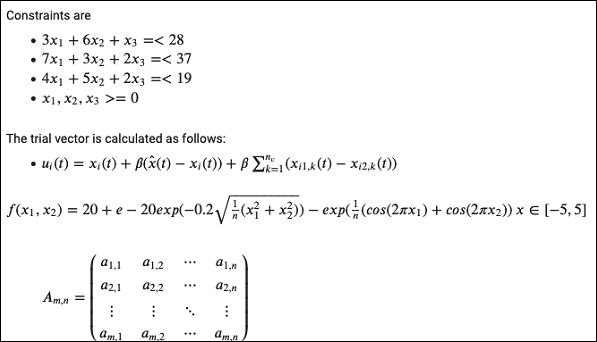As the code cell supports full Python syntax, you may use Python comments in the code window to describe your code. However, many a time you need more than a simple text based comments to illustrate the ML algorithms. ML heavily uses mathematics and to explain those terms and equations to your readers you need an editor that supports LaTex - a language for mathematical representations. Colab provides Text Cells for this purpose.

A text cell containing few mathematical equations typically used in ML is shown in the screenshot below −As we move ahead in this chapter, we will see the code for generating the above output.

Text Cells are formatted using markdown - a simple markup language. Let us now see you how to add text cells to your notebook and add to it some text containing mathematical equations.

## Markdown Examples

Let us look into few examples of markup language syntax to demonstrate its capabilities.

Type in the following text in the Text cell.

This is **bold**.
This is *italic*.
This is ~strikethrough~.


The output of the above commands is rendered on the right hand side of the Cell as shown here.## Mathematical Equations

Add a Text Cell to your notebook and enter the following markdown syntax in the text window −

$\sqrt{3x-1}+(1+x)^2$


You will see the immediate rendering of the markdown code in the right hand side panel of the text cell. This is shown in the screenshot below −Hit Enter and the markdown code disappears from the text cell and only the rendered output is shown.

Let us try another more complicated equation as shown here −

$e^x = \sum_{i = 0}^\infty \frac{1}{i!}x^i$


The rendered output is shown here for your quick reference.## Code for Sample Equations

Here is the code for the sample equations shown in an earlier screenshot −

Constraints are
- $3x_1 + 6x_2 + x_3 =< 28$
- $7x_1 + 3x_2 + 2x_3 =< 37$
- $4x_1 + 5x_2 + 2x_3 =< 19$
- $x_1,x_2,x_3 >=0$

The trial vector is calculated as follows:
- $u_i(t) = x_i(t) + \beta(\hat{x}(t) − x_i(t)) + \beta \sum_{k = 1}^{n_v}(x_{i1,k}(t) − x_{i2,k}(t))$
$f(x_1, x_2) = 20 + e - 20exp(-0.2 \sqrt {\frac {1}{n} (x_1^2 + x_2^2)}) - exp (\frac {1}{n}(cos(2\pi x_1) + cos(2\pi x_2))$

$x ∈ [-5, 5]$
>$A_{m,n} = \begin{pmatrix} a_{1,1} > a_{1,2} > \cdots > a_{1,n} \\ a_{2,1} > a_{2,2} > \cdots > a_{2,n} \\ \vdots > \vdots > \ddots > \vdots \\ a_{m,1} > a_{m,2} > \cdots > a_{m,n} \end{pmatrix}$


Describing full markup syntax is beyond the scope of this tutorial. In the next chapter, we will see how to save your work.